# Chapter 3 T-Tests

## 3.1 Independent Samples T-Test

An example from Hays (1974, pp. 404-407):

"An experimenter working in the area of motivational factors in perception was interested in the effects of deprivation upon the perceived size of objects. Among the studies carried out was one done with orphans, who were compared with nonorphaned children on the basis of the judged size of parental figures viewed at a distance. […]Now two independent randomly selected groups were used. Sample 1 was a group of orphaned children without foster parents. Sample 2 was a group of children having a normal family with both parents. Both population of children sampled showed the same age level, sex distribution, educational level, and so forth. The question asked by the experimenter was ‘Do deprived children tend to judge the parental figures relatively larger than do the nondeprived?’ In terms of a null and alternative hypothesis,

H0: $$\mu$$1 - $$\mu$$2 $$\le 0$$

H1: $$\mu$$1 - $$\mu$$2 $$> 0$$.

The $$\alpha$$ level for significance decided upon was .05. The actual results were

Sample 1:

M1 = 1.8

S1 = .7

N1 = 125

Sample 2:

M2 = 1.6

S2 = .9

N2 = 150"

Note: A data set with these properties has been simulated using R.

### 3.1.1 Results Overview

Table 3.1: Result Overview Independent Samples T-Test
By Hand JASP SPSS SAS Minitab R
t (Welch) 2.11 2.0717 2.072 2.07 2.07 2.0717
t (Student) NA 2.0257 2.026 2.03 2.03 2.0257

### 3.1.2 By Hand

Calculations by hand can be found in Hays, 1974, pp. 404-407.

Result: t = 2.11

Significant (two-tailed test) for $$\alpha$$ = .05 or less

Note: Hays calculated only the Welch T-test.

### 3.1.3 JASP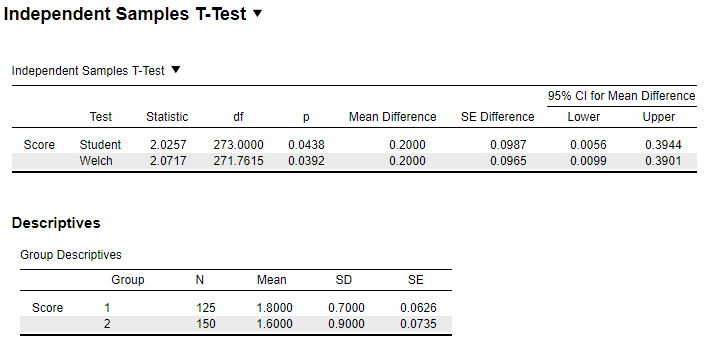Figure 3.1: JASP Output for Independent Samples T-Test

### 3.1.4 SPSS

DATASET ACTIVATE DataSet1.
T-TEST GROUPS=groups(1 2)
/MISSING=ANALYSIS
/VARIABLES=samples
/CRITERIA=CI(.95).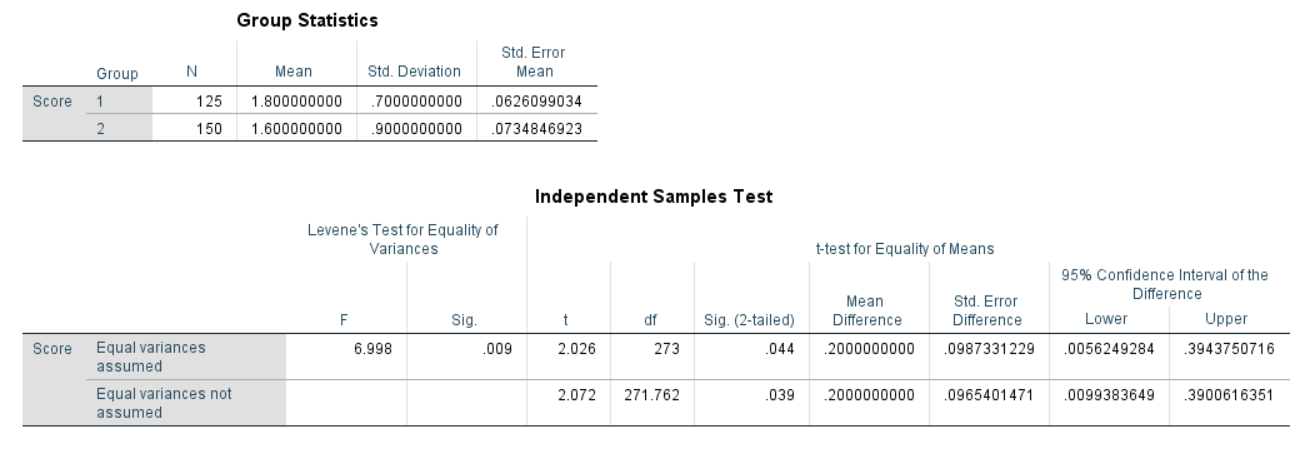Figure 3.2: SPSS Output for Independent Samples T-Test

### 3.1.5 SAS

proc ttest data=istt sides=2 alpha=0.05 h0=0;
title "Two sample t-test";
class Group;
var Score;
run;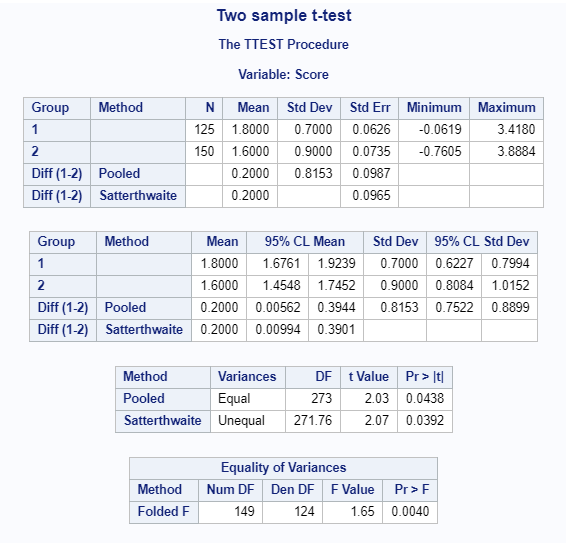Figure 3.3: SAS Output for Independent Samples T-Test

### 3.1.6 Minitab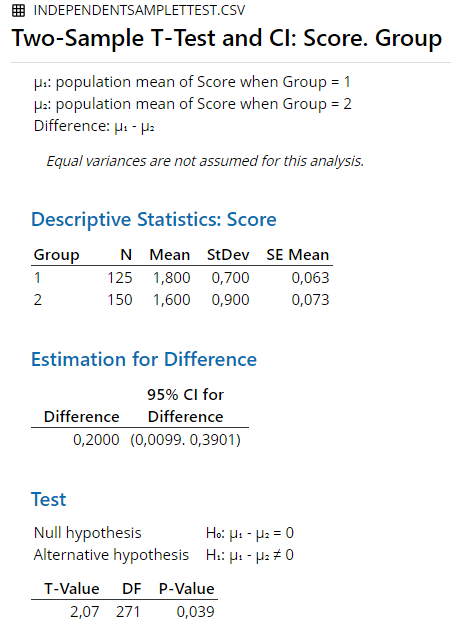Figure 3.4: Minitab Output for Welch Independent Samples T-Test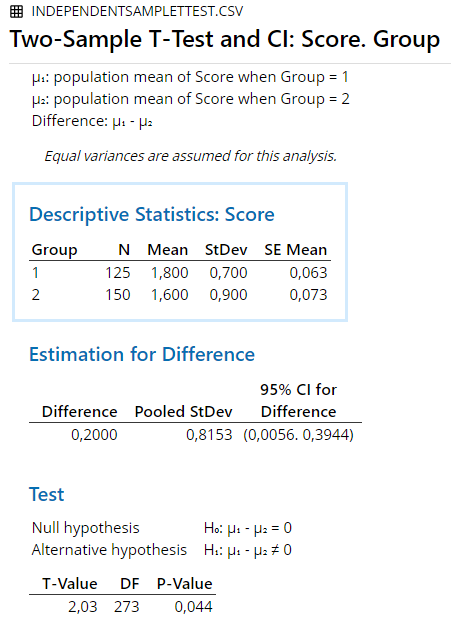Figure 3.5: Minitab Output for Student Independent Samples T-Test

### 3.1.7 R

t.test(subset(istt.data, Group == 1)$Score, subset(istt.data, Group == 2)$Score)
##
## 	Welch Two Sample t-test
##
## data:  subset(istt.data, Group == 1)$Score and subset(istt.data, Group == 2)$Score
## t = 2.0717, df = 271.76, p-value = 0.03924
## alternative hypothesis: true difference in means is not equal to 0
## 95 percent confidence interval:
##  0.009938365 0.390061635
## sample estimates:
## mean of x mean of y
##       1.8       1.6
t.test(subset(istt.data, Group == 1)$Score, subset(istt.data, Group == 2)$Score, var.equal = T)
##
## 	Two Sample t-test
##
## data:  subset(istt.data, Group == 1)$Score and subset(istt.data, Group == 2)$Score
## t = 2.0257, df = 273, p-value = 0.04377
## alternative hypothesis: true difference in means is not equal to 0
## 95 percent confidence interval:
##  0.005624928 0.394375072
## sample estimates:
## mean of x mean of y
##       1.8       1.6

### 3.1.8 Remarks

All differences in results between the software and hand calculation are due to rounding.

### 3.1.9 References

Hays, W. L. (1974). Statistics for the social sciences (2nd Ed.). New York, US: Holt, Rinehart and Winston, Inc.

## 3.2 Mann-Whitney Test

An example from Hays (1974, pp. 778-780):

“As an example, consider the following data:”

Table 3.2: Data for Mann-Whitney Test
Score Group
8 A
3 A
4 A
6 A
1 B
7 B
9 B
10 B
12 B

### 3.2.1 Results Overview

Table 3.3: Result Overview Mann-Whitney Test
By Hand JASP SPSS SAS Minitab R
U’ 5 5 5 5 5 5

### 3.2.2 By Hand

Calculations by hand can be found in Hays, 1974, pp. 778-780.

Result:

U’ = 5

U = 15

Not significant for $$\alpha$$ = .05 or less.

### 3.2.3 JASPFigure 3.6: JASP Output for Mann-Whitney Test

Note: W corresponds to U’ in the hand calculation.

### 3.2.4 SPSS

DATASET ACTIVATE DataSet1.
*Nonparametric Tests: Independent Samples.
NPTESTS
/INDEPENDENT TEST (Score) GROUP (Group) MANN_WHITNEY
/MISSING SCOPE=ANALYSIS USERMISSING=EXCLUDE
/CRITERIA ALPHA=0.05  CILEVEL=95.Figure 3.7: SPSS Output for Mann-Whitney Test

Note: U = 15 corresponds to U’ = 5.

### 3.2.5 SAS

PROC npar1way data=work.mwt wilcoxon;
class Group;
var Score;
run;Figure 3.8: SAS Output for Mann-Whitney Test

Note: U = 15 corresponds to U’ = 5.

### 3.2.6 MinitabFigure 3.9: Minitab Output for Mann-Whitney Test

Note: W corresponds to U in the hand calculation and U = 15 corresponds to U’ = 5.

### 3.2.7 R

wilcox.test(Score~Group, data = MWT.data2)
##
## 	Wilcoxon rank sum exact test
##
## data:  Score by Group
## W = 5, p-value = 0.2857
## alternative hypothesis: true location shift is not equal to 0

Note: W corresponds to U’ in the hand calculation.

### 3.2.8 Remarks

All differences in results between the software and hand calculation are due to rounding.

The output for the Mann-Whitney test is not clearly defined, leading to different conventions in different software. The R documentation explains it as follows:

“The two most common definitions correspond to the sum of the ranks of the first sample with the minimum value subtracted or not: R subtracts and S-PLUS does not, giving a value which is larger by m(m+1)/2 for a first sample of size m. (It seems Wilcoxon’s original paper used the unadjusted sum of the ranks but subsequent tables subtracted the minimum.) R’s value can also be computed as the number of all pairs (x[i], y[j]) for which y[j] is not greater than x[i], the most common definition of the Mann-Whitney test.”

### 3.2.9 References

Hays, W. L. (1974). Statistics for the social sciences (2nd Ed.). New York, US: Holt, Rinehart and Winston, Inc.

## 3.3 Paired Samples T-Test

An example from Hays (1974, pp. 424-427):

“Consider once again the question of scores on a test of dominance. The basic question has to do with the mean score for men as opposed to the mean score for women. In carrying out the experiment, the investigator decided to sample eight husband-wife-pairs at random. The members of each pair were given the test of dominance separately, and the data turned out as follows:”

Table 3.4: Data for Paired Sample T-Test
Husband Wife
26 30
28 29
28 28
29 27
30 26
31 25
34 24
37 23

### 3.3.1 Results Overview

Table 3.5: Result Overview Paired Samples T-Test
By Hand JASP SPSS SAS Minitab R
t 1.838 1.8381 1.838 1.84 1.84 1.8381

### 3.3.2 By Hand

Calculations by hand can be found in Hays, 1974, pp. 424-427.

Result: t = 1.838

Not significant (two-tailed test) for $$\alpha$$ = .05 or less ### JASP {#jaspPSTT}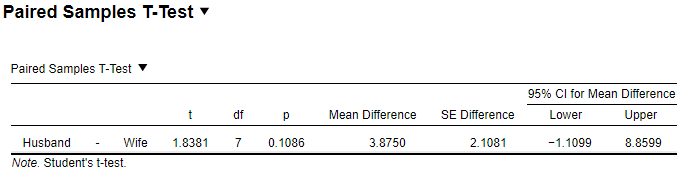Figure 3.10: JASP Output for Paired Samples T-Test

### 3.3.3 SPSS

DATASET ACTIVATE DataSet1.
T-TEST PAIRS=Husband WITH Wife (PAIRED)
/CRITERIA=CI(.9500)
/MISSING=ANALYSIS.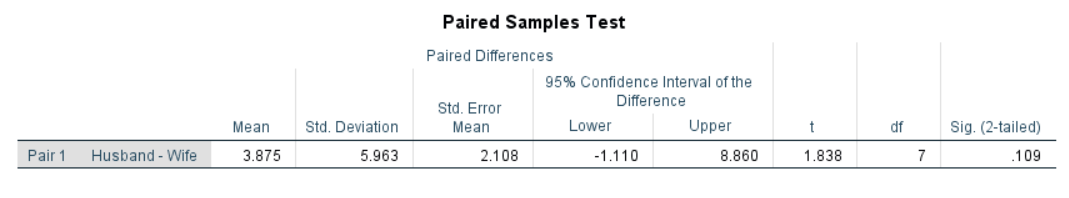Figure 3.11: SPSS Output for Paired Samples T-Test

### 3.3.4 SAS

proc ttest data=pstt sides=2 alpha=0.05 h0=0;
title "Paired sample t-test";
paired Husband * Wife;
run;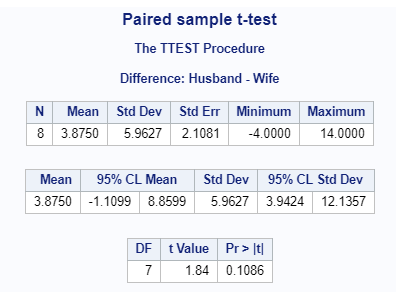Figure 3.12: SAS Output for Paired Samples T-Test

### 3.3.5 Minitab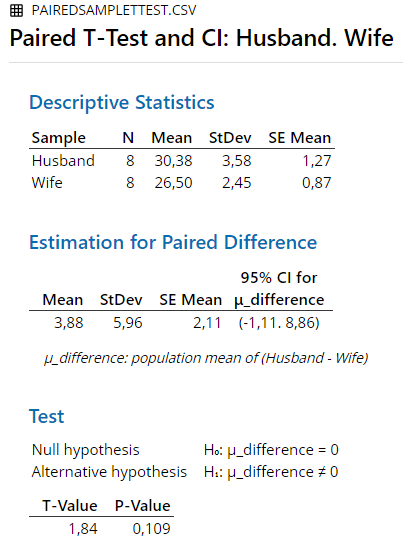Figure 3.13: Minitab Output for Paired Samples T-Test

### 3.3.6 R

t.test(pstt.data$Husband, pstt.data$Wife, paired = T)
##
## 	Paired t-test
##
## data:  pstt.data$Husband and pstt.data$Wife
## t = 1.8381, df = 7, p-value = 0.1086
## alternative hypothesis: true difference in means is not equal to 0
## 95 percent confidence interval:
##  -1.109927  8.859927
## sample estimates:
## mean of the differences
##                   3.875

### 3.3.7 Remarks

All differences in results between the software and hand calculation are due to rounding.

### 3.3.8 References

Hays, W. L. (1974). Statistics for the social sciences (2nd Ed.). New York, US: Holt, Rinehart and Winston, Inc.

## 3.4 Wilcoxon Test

An example from Hays (1974, pp. 780-781):

“Suppose that in some experiment involving a single treatment and one control group, subjects were first matched pairwise, and then one member of each pair was assigned to the experimental group at random. In the experiment proper, each subject received some Y score.”

Table 3.6: Data for Wilcoxon Test
Treatment. Control
83 75
80 78
81 66
74 77
79 80
78 68
72 75
84 90
85 81
88 83

### 3.4.1 Results Overview

Table 3.7: Result Overview Wilcoxon Test
By Hand JASP SPSS SAS Minitab R
W’ 15 15 15 NA 15 15

### 3.4.2 By Hand

Calculations by hand can be found in Hays, 1974, pp. 780-781.

Result:

T = 15

Not significant for $$\alpha$$ = .05 or less.

### 3.4.3 JASP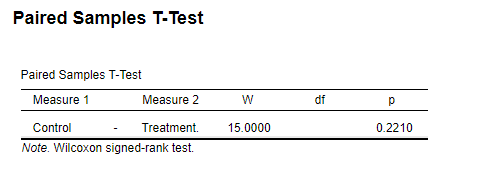Figure 3.14: JASP Output for Wilcoxon Test

### 3.4.4 SPSS

DATASET ACTIVATE DataSet1.
*Nonparametric Tests: Related Samples.
NPTESTS
/RELATED TEST(Treatment Control) WILCOXON
/MISSING SCOPE=ANALYSIS USERMISSING=EXCLUDE
/CRITERIA ALPHA=0.05  CILEVEL=95.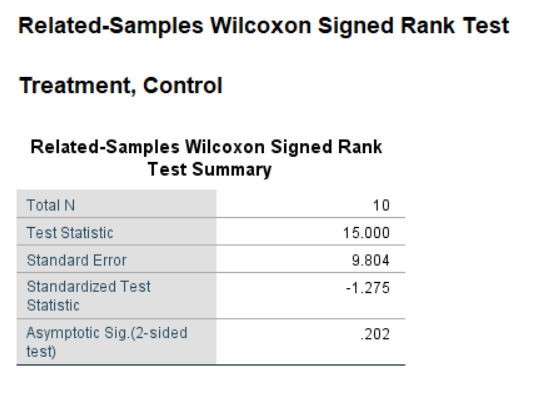Figure 3.15: SPSS Output for Wilcoxon Test

### 3.4.5 SAS

Not available in SAS.

### 3.4.6 Minitab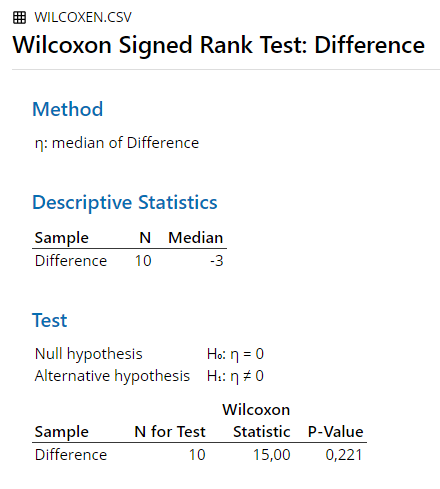Figure 3.16: Minitab Output for Wilcoxon Test

### 3.4.7 R

wilcox.test(Wilcoxon.data2$Control, Wilcoxon.data2$Treatment., paired = T)
## Warning in wilcox.test.default(Wilcoxon.data2$Control, ## Wilcoxon.data2$Treatment., : cannot compute exact p-value with ties
##
## 	Wilcoxon signed rank test with continuity correction
##
## data:  Wilcoxon.data2$Control and Wilcoxon.data2$Treatment.
## V = 15, p-value = 0.221
## alternative hypothesis: true location shift is not equal to 0

### 3.4.8 Remarks

All differences in results between the software and hand calculation are due to rounding.

### 3.4.9 References

Hays, W. L. (1974). Statistics for the social sciences (2nd Ed.). New York, US: Holt, Rinehart and Winston, Inc.

## 3.5 One Sample T-Test

A dataset with the following properties has been generated using R:

n = 100

M = 5

$$\sigma$$ = 1

The expected mean to perform the One Sample T-test against is:

E(M) = 3

The alpha level was chosen to be:

$$\alpha$$ = .05

### 3.5.1 Results Overview

Table 3.8: Result Overview One Sample T-Test
By Hand JASP SPSS SAS Minitab R
t 20 20 20 20 20 20

### 3.5.2 By Hand

t = $$\frac{M - E(M) }{\sigma/\sqrt{n}}$$

therefore:

t = $$\frac{5-3}{1/\sqrt{100}}$$ = $$\frac{2}{1/10}$$ = 20

A t-score of 20 is significant for a two tailed test at $$\alpha$$ = .05 or less.

### 3.5.3 JASP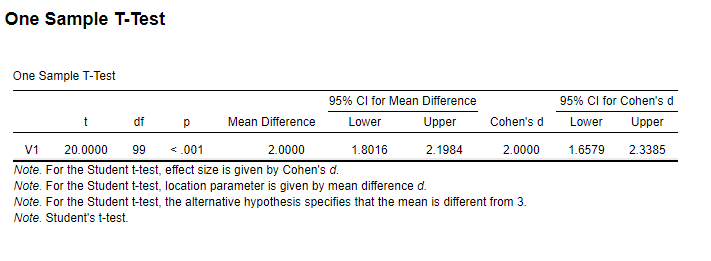Figure 3.17: JASP Output for One Sample T-Test

### 3.5.4 SPSS

DATASET ACTIVATE DataSet1.
T-TEST
/TESTVAL=3
/MISSING=ANALYSIS
/VARIABLES=V1
/CRITERIA=CI(.95).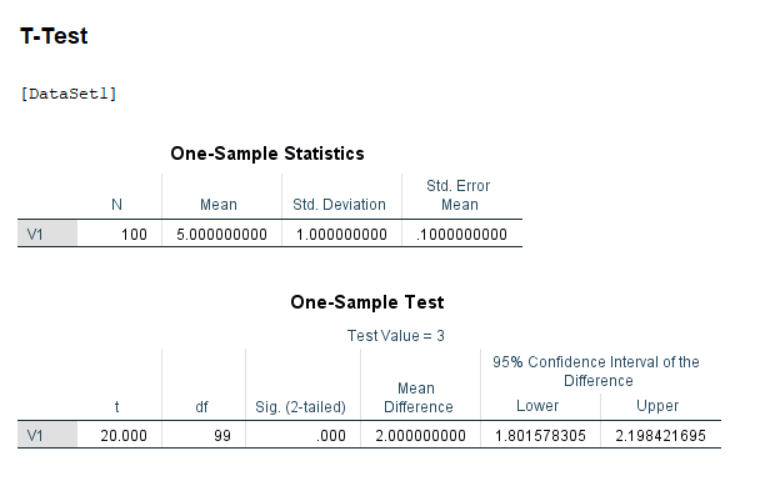Figure 3.18: SPSS Output for One Sample T-Test

### 3.5.5 SAS

proc ttest data=work.OSTT sides=2 alpha=0.05  h0=3;
var Data;
run;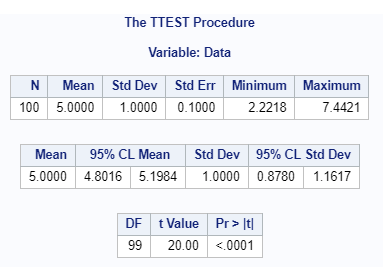Figure 3.19: SAS Output for One Sample T-Test

### 3.5.6 Minitab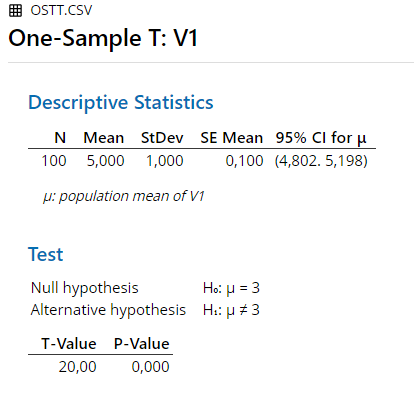Figure 3.20: Minitab Output for One Sample T-Test

### 3.5.7 R

t.test(ostt.data2$V1, mu = 3) ## ## One Sample t-test ## ## data: ostt.data2$V1
## t = 20, df = 99, p-value < 2.2e-16
## alternative hypothesis: true mean is not equal to 3
## 95 percent confidence interval:
##  4.801578 5.198422
## sample estimates:
## mean of x
##         5

### 3.5.8 Remarks

All differences in results between the software and hand calculation are due to rounding.